Courses

# Test: Determinacy & Indeterminacy -1

## 10 Questions MCQ Test Topicwise Question Bank for Civil Engineering | Test: Determinacy & Indeterminacy -1

Description
This mock test of Test: Determinacy & Indeterminacy -1 for GATE helps you for every GATE entrance exam. This contains 10 Multiple Choice Questions for GATE Test: Determinacy & Indeterminacy -1 (mcq) to study with solutions a complete question bank. The solved questions answers in this Test: Determinacy & Indeterminacy -1 quiz give you a good mix of easy questions and tough questions. GATE students definitely take this Test: Determinacy & Indeterminacy -1 exercise for a better result in the exam. You can find other Test: Determinacy & Indeterminacy -1 extra questions, long questions & short questions for GATE on EduRev as well by searching above.
QUESTION: 1

### Total degree of indeterminacy (both internal and external) of the plane frame shown in the given figure is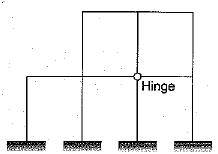Solution:

The degree of indeterminacy,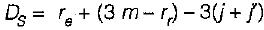Number of external reactions = re
= 3 + 3 + 3 + 3 = 12
Number of rigid joints,
j= 10
Number of joints at which releases are located,
j= 1
Number of members,
m = 12
As the hinge is located at a point where 4 members meet. Hence it is equivalent to three hinges.
Therefore number of releases, r= 3.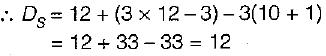QUESTION: 2

### If there are m unknown member forces, r unknown reaction components and j number of joints, then the degree of static indeterminacy of a pin-jointed plane frame is given by​

Solution:

Ds for a pin jointed frame = m+r-2j
Ds for a rigid jointed frame = 3m+r -3j
m- No. of members, r- no. of reactions, j- no. of joints

QUESTION: 3

### Degree of kinematic indeterminacy of a pin jointed plane frame is given by where j is number of joints and r is reaction components.

Solution:

Dk = 2j –r for a pin jointed frame
Dk = 3j –r for a rigid jointed frame
j number of joints and r number of reactions.

QUESTION: 4

A pin-jointed plane frame is unstable if where m is number of members r is reaction components and j is number of joints

Solution:
QUESTION: 5

The degree of static indeterminacy of a pin- jointed space frame is given by

Solution:
QUESTION: 6

The degree of kinematic indeterminacy of a pin-jointed space frame is given by

Solution:
QUESTION: 7

The degree of static indeterminacy of the pin- jointed plane frame shown in figure is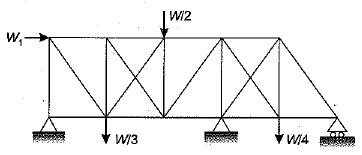Solution:

External indeterminacy,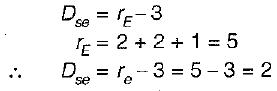Internal indeterminacy,No, of members, m = 21
Number of joints, j = 11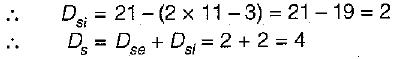QUESTION: 8

The portal frame as shown in the given frame is statically indeterminate to the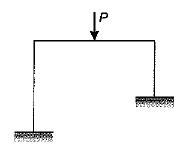Solution:

m=3; r= 3+3 =6 ; j=4
Ds = 3m+r-3j = 3(3) + 6 -3(4) = 3

QUESTION: 9

A perfect plane frame having n number of members and j number of joints should satisfy the relation​

Solution:

A perfect plane frame means a determinate structure, so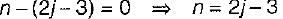QUESTION: 10

Neglecting axial deformation, the kinematic indeterminacy of the structure shown in the figure below is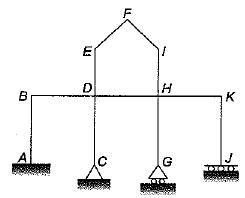Solution:

j = 11; r=8; m=11
Dk = 3j-r-no. of inextensible members = 3(11) – 8 -11 = 14